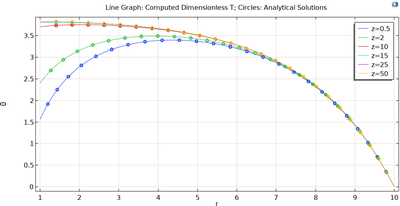# Galleria delle Applicazioni

## Bioheat Transfer verification

Application ID: 106561

In this model, we use the Bioheat Transfer interface to solve the Pennes bioheat transfer equation. The problem is in 1D and formulated to be dimensionless. The results are compared against analytical solutions derived in a journal paper (Ref. 1). A mesh convergence study shows that the computed solution converges to the analytical solution as number of elements increases. The model serves as a good verification for the Bioheat Transfer interface.

 Huang, H. W.; Chan, C. L.; Roemer, R. B. Analytical Solutions of Pennes Bio-Heat Transfer Equation With a Blood Vessel. Journal of Biomechanical Engineering 1994, 116 (2), 208–212. https://doi.org/10.1115/1.2895721.This model example illustrates applications of this type that would nominally be built using the following products: